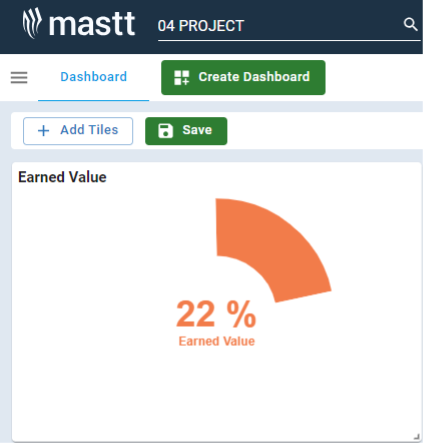# Earned Value

Earned Value is a crucial metric in capital project cost management. It is the quantification of the work actually accomplished at a given point in the project, translated into the budgeted cost of that work.Calculating Earned Value (EV) on Capital Projects involves three essential metrics: Planned Value (PV), Actual Cost (AC), and Earned Value itself. The process requires measuring the project's performance at a specific point in time.

1. Planned Value (PV): This is the budgeted cost for the work scheduled to be completed at a given point in time. To calculate PV, multiply the planned percentage of completed work by the total project budget (BAC): PV = (Planned % Complete) x (BAC).
2. Actual Cost (AC): This is the actual money spent on work completed so far. It's also known as the actual cost of work performed (ACWP).
3. Earned Value (EV): To calculate EV, you need to quantify the amount of work completed so far and convert it into its corresponding budgeted cost. This can be done by multiplying the percentage of completed work by the total project budget (BAC): EV = (Actual % Complete) x (BAC).

By calculating these metrics, you can effectively assess the performance of your capital projects, analyze variances, and make informed decisions.